NEET  >  NCERT Exemplars: Semiconductor Electronics - 2

# NCERT Exemplars: Semiconductor Electronics - 2 - Notes | Study Physics Class 12 - NEET

 1 Crore+ students have signed up on EduRev. Have you?

Q.17. Why are elemental dopants for Silicon or Germanium usually chosen from group XIII or group XV?
Ans.
When pure semiconductor material is mixed with small amounts of certain specific impurities with valency different from that of the parent material, the number of mobile electrons/holes drastically changes. The process of addition of impurity is called doping. The size of the dopant atom should be compatible such that their presence in the pure semiconductor does not distort the semiconductor but easily contribute the charge carriers on forming covalent bonds with Silicon or Germanium atoms, which are provided by group XIII or group XV elements.

Q.18. Sn, C, and Si, Ge are all group XIV elements. Yet, Sn is a conductor, C is an insulator while Si and Ge are semiconductors. Why?
Ans.
The conduction level of any element depends on the energy gap between its conduction band and valence band.
In conductors, there is no energy gap between conduction band and valence band. For insulator, the energy gap is large and for semiconductor the energy gap is moderate.
The energy gap for Sn is 0 eV, for C is 5.4 eV, for Si is 1.1 eV and for Ge is 0.7 eV related to their atomic size. Therefore Sn is a conductor, C is an insulator, and Ge and Si are semiconductors

Q.19. Can the potential barrier across a p-n junction be measured by simply connecting a voltmeter across the junction?
Ans. We cannot measure the potential barrier across a p-n junction by a voltmeter because the resistance of voltmeter is very high as compared to the junction resistance. Potential of potential barrier for Ge is VB = 0.3 V and for silicon is VB = 0.7 V.
On the average the potential barrier in P-N junction is ~0.5 V.

Q.20. Draw the output waveform across the resistor (Figure).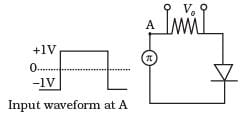Ans.
The diode act as a half wave rectifier, it offers low resistance when forward biased and high resistance when reverse biased. So the output is obtained only when positive input is given,so the output waveform is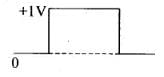Q.21. The amplifiers X, Y and Z are connected in series. If the voltage gains of X, Y and Z are 10, 20 and 30, respectively and the input signal is 1 mV peak value, then what is the output signal voltage (peak value)
(i) if dc supply voltage is 10V?
(ii) if dc supply voltage is 5V?
Ans.
Total voltage amplification is defined as the ratio of output signal voltage and input signal voltage.
According to the problem, voltage gain in X, vx = 10,
voltage gain in Y; vy = 20,
voltage gain in Z, vz, = 30;
ΔV= 1 mV = 10-3 V
And Total voltage amplification = vx * vy * vz,
ΔV0 = v* vy * vz * ΔVi
= 10 *20 * 30 * 10-3 = 6V
(i) If DC supply voltage is 10 V, then output is 6 V, since theoretical gain is equal to practical gain, i.e., output can never be greater than 6 V.
(ii) If DC supply voltage is 5 V, i.e., Vcc = 5 V. Then, output peak will not exceed 5 V. Hence V0 = 5 V.

Q.22. In a CE transistor amplifier there is a current and voltage gain associated with the circuit. In other words there is a power gain.
Considering power a measure of energy, does the circuit violate  conservation of energy?
Ans.

Key Concept: Different gain in CE transistor amplifier.
(i) ac current  gain: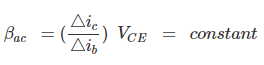(ii) dc current gain :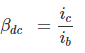(iii) Voltage gain: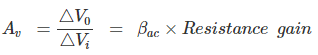(iv) Power gain: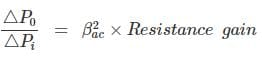The power gain is very high in CE transistor amplifier. In this circuit, the extra power required for amplified output is obtained from DC source. Thus, the circuit used does not violate the law of conservation.

Q.23.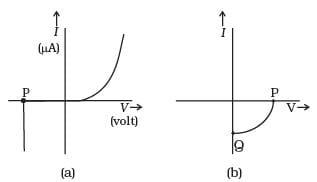(i) Name the type of a diode whose characteristics are shown in Figure (A) and Fig. (B).
(ii) What does the point P in Figure (A) represent?
(iii) What does the points P and Q in Figure (B) represent?
Ans.
(i) Figure (a) represents the characteristics of Zener diode and curve (b) is of solar cell.
(ii) In figure (a), point P represents Zener breakdown voltage.
(iii) In figure (b), the point Q represents zero voltage and negative current. Which means the light falling on solar cell with atleast minimum threshold frequency gives the current in opposite direction to that due to a battery connected to solar cell. But for the point Q the battery is short circuited. Hence it represents the short circuit current.
And the point Pin figure (b) represents some open circuit, voltage on solar cell with zero current through solar cell.
It means, there is a battery connected to a solar cell which gives rise to the equal and opposite current to that in solar cell by virtue of light falling on it.

Q.24. Three photo diodes D1, D2 and D3 are made of semiconductors having band gaps of 2.5 eV, 2 eV and 3 eV, respectively. Which ones will be able to detect light of wavelength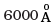?
Ans.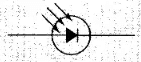According to the problem,
Wavelength of light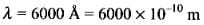energy of the light photon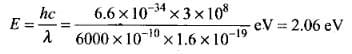The incident radiation which is detected by the photodiode D2 because energy of incident radiation is greater than the band-gap.

Q.25. If the resistance R1 is increased (Figure), how will the readings of the ammeter and voltmeter change?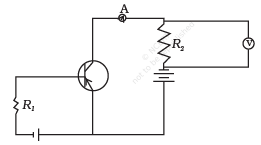Ans.
Let us redrawn the circuit diagram to find the change in reading of ammeter and voltmeter.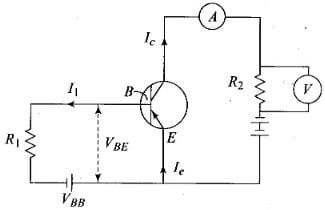so, IBR1 + VBE = VBB
Basic current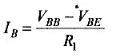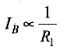So, R1 is increased, IB is decreased.
Now, the current in ammeter is collector current IC.
IC = βIB as IB is decreased, IC is also decreased and the reading of voltmeter and ammeter also decreased.

Q.26. Two car garages have a common gate which needs to open automatically when a car enters either of the garages or cars enter both. Devise a circuit that resembles this situation using diodes for this situation.
Ans.
As car enters in either of the garages or both, the common gate opened automatically.
This means that if any one input is high, output will high otherwise low.
The device is shown like this: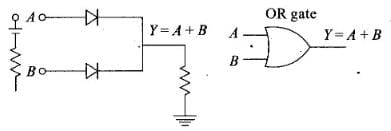So, OR gate gives the desired output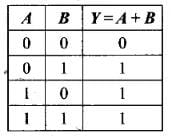Q.27. How would you set up a circuit to obtain NOT gate using a transistor?
Ans.

(1) It has only one input and only one output.
(2) Boolean expression is Y = Ᾱ and is read as “y equals not A” .
Logical symbol of NOT gate.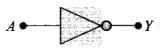(3) Realization of NOT gate: The transistor is so biased that the collector voltage VCC = V (Voltage corresponding to 1 state)
The resistors R and RB are so chosen that if the input is low, i.e. 0, the transistor is in the cut off and hence the voltage appearing at the output will be the same as applied V = 5 V. Hence Y = V(or state I)
If the input is high, the transistor current is in saturation and the net voltage at the output Y is 0 (in state 0)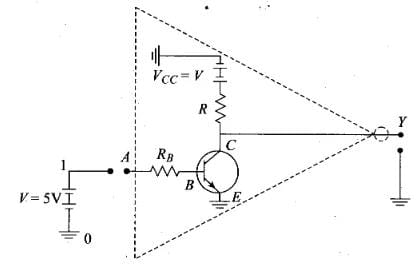(4) Truth table for Not gate: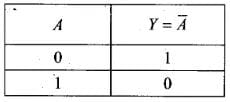Q.28. Explain why elemental semiconductor cannot be used to make visible LEDs.
Ans.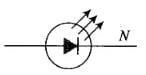Specially designed diodes, which give out light radiations when forward biases. LED 's are made of GaAsp, Gap etc.
These are forward biased P-N junctions which emits spontaneous radiation.
In elemental semiconductor, the band gap is such that the emission arc in infrared region and not in visible region.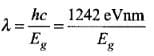for Si; Eg = 1.1 eV, λ = 1242/1.1 = 1129 nm
for Ge; Eg = 0.7 eV, λ = 1242/0.7 = 1725 nm

Q.29. Write the truth table for the circuit shown in Figure. Name the gate that the circuit resembles.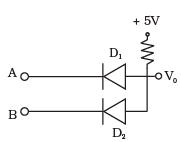Ans.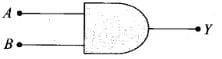Logical Symbol of AND gateThis is 'AND' Gate and its characteristics are as follows:
(1) it has two inputs (A and B) and only one output (Y)
(2) Boolean expression is Y = A.B is read as "Y equals A AND B"
(3) Realization of AND gate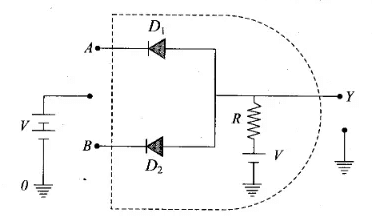(i) A = 0 , B = 0
The voltage supply through R is forward biasing diodes D1 and D2 (offers low resistance), the voltage V would drop across R.
The output voltage at Y= the voltage across diode = 0
(ii) A = 0, B = I
D1 = Conducts, D2 = Not Conducts
The out voltage at Y= The voltage across the diode (D2) = 0
(iii) A = 1 , B = 0
D1 = Conducts, D2 = Not conducts
The out voltage at Y= The voltage across the diode (D2) = 0
(iv) A = 1 , B = 1
None of the diode conducts
The out voltage at Y= Battery voltage = I
(4) Truth table for ‘AND' gate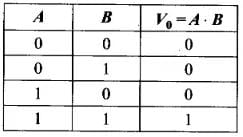Q.30. A Zener of power rating 1 W is to be used as a voltage regulator. If zener has a breakdown of 5V  and it has to regulate voltage which fluctuated between 3V and 7V, what should be the value of Rs for safe operation (Figure)?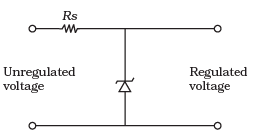Ans.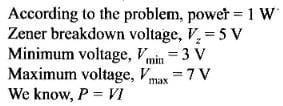According to the problem power = 1 W
Zener breakdown voltage, Vz = 5 V
Minimum voltage, Vmin = 3 V
Maximum voltage, Vmax = 7 V
We know, P = VIfor safe operations Rs will be equal to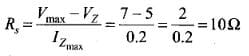Q.31. If each diode in Figure has a forward bias resistance of 25Ω and infinite resistance in reverse bias, what will be the values of the current I1, I2, I3 and I4?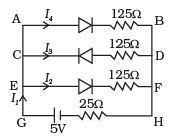Ans.

According to the problem, forward biased resistance = 25 Ω and reverse biased resistance = ∞.
As shown in the figure, the diode in branch CD is in reverse biased which having infinite resistance.
So, current in that branch is zero, i.e. I3 = 0
Resistance in branch AB = 25 + 125 = 150 Ω, say R1
Resistance in branch EF - 25 + 125 = 150 Ω, say R2
AB is parallel to EF.
So, effective resistance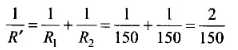⇒ R' = 75Ω
Total resistance R of the circuit - R' + 25 = 75 + 25 = 100 U.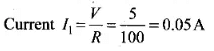According to the kirchoff’s, current law (K.CL),
I1=I4 + I2 + I3   (Here I3 = 0)
So, I= I4 + I2
Here, the resistances R1 and R2 is same.
i.e., I4 = I2
∴  I1 = 2I2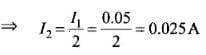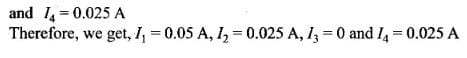and I4 = 0.025 A
Therefore, we get, I1, = 0.05 A, I2 = 0.025 A, I3 = 0 and I4 = 0.025 A

Q.32. In the circuit shown in Figure when the input voltage of the base resistance is 10 V, Vbe is zero and Vce is also zero. Find the values of Ib, Ic and β.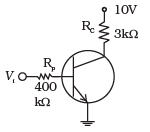Ans.
Voltage across RB = 10V
V= RBIB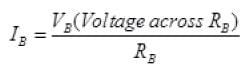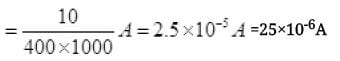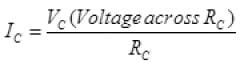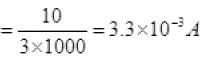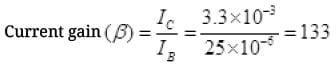Q.33 Draw the output signals C1 and C2 in the given combination of gates (Figure).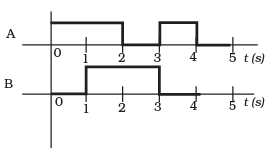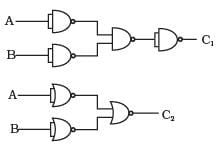Ans.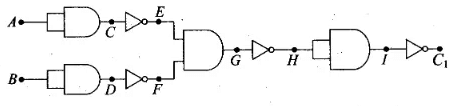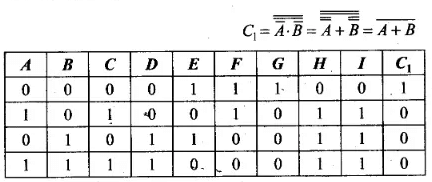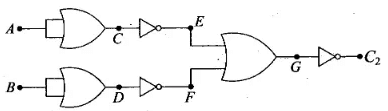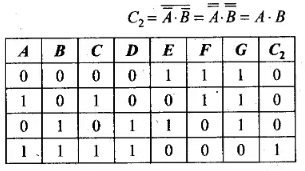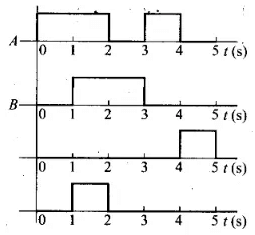Q.34. Consider the circuit arrangement shown in Figure (a) for studying input and output characteristics of npn transistor in CE configuration.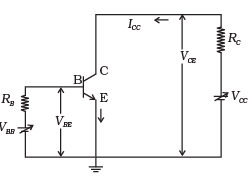(a)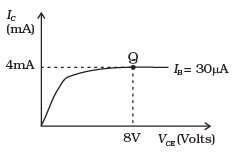(b)

Select the values of RB and RC for a transistor whose VBE = 0.7 V, so that the transistor is operating at point Q as shown in the characteristics shown in Figure (b).
Given that the input impedance of the transistor is very small and VCC = VBB = 16 V, also find the voltage gain and power gain of circuit making appropriate assumptions.
Ans.
For output characteristic at point Q
VCE = 8V, I= 30 μA, I= 4 mA, VBE = 0.7V
Applying Kirchhoff’s law in collector-emitter loop
VCC=VCE+RCIC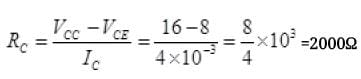RC= 2000Ω
Now applying Kirchhoff’s loop law in base-emitter circuit,
VBB=IBR+ VBE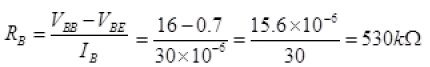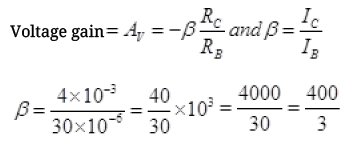Average Voltage AV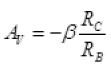(-) sign shows change in phase angle of output is by input voltage.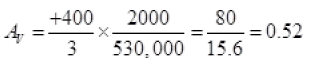Power gain = I.V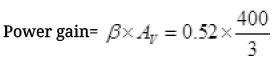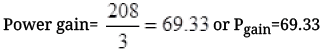Q.35. Assuming the ideal diode, draw the output waveform for the circuit given in Figure Explain the waveform.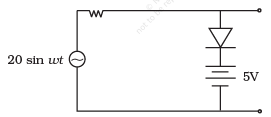Ans.
Key concept: An ideal diode is a diode in which it has a very large resistance in reverse biased and very, low resistance in forward biased. So, it acts like a perfect conductor when voltage is applied forward biased and like a perfect insulator when voltage is applied reverse biased.
In reverse biased when the input voltage is equal to or less than 5 V diode,then it will offer high resistance in comparison to resistance (R) in series. Now, diode appears in open circuit. The input waveform is then passed to the output terminals. The result with sin wave input is to dip off all positive going portion above 5 V.
If input voltage is greater than +5 V, diode is in conducting state, then it will be conducting as if forward biased offering low resistance in comparison to R. But there will be no voltage in output beyond 5 V as the voltage beyond +5 V will appear across R.
When input voltage is negative, there will be opposition to 5 V battery in p-n junction input voltage becomes more than -5 V, the diode will be reverse biased. It will offer high resistance in comparison to resistance R in series. Now junction diode appears in open circuit. The input wave form is then passed on to the output terminals.
The output waveform will be like this (as shown below).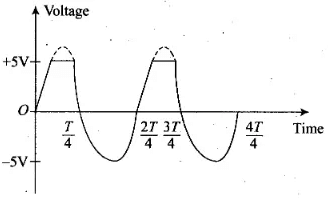Q.36. Suppose a ‘n’-type wafer is created by doping Si crystal having 5 × 1028 atoms/m3 with 1ppm concentration of As. On the surface 200 ppm Boron is added to create ‘P’ region in this wafer. Considering nt = 1.5 × 1016 m–3,
(i) Calculate the densities of the charge carriers in the n & p regions.
(ii) Comment which charge carriers would contribute largely for the reverse saturation current when diode is reverse biased.
Ans.

When As (pentavalent) is added to Si the n-type water is created.
So the number of majority carriers in n-type water,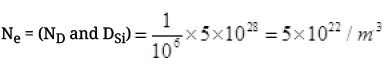For number of minority carriers nh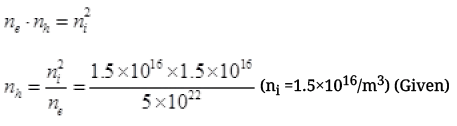=0.3 × 1.5 × 1032-22 = 0.45 × 10+10    perm3
When boron(trivalent) is implanted in Si crystal, p- type wafer is formed with number of holes,
nh = (ND × n of Si)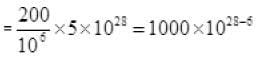nh = 1×1025 per m3
Minority carrier in p-type water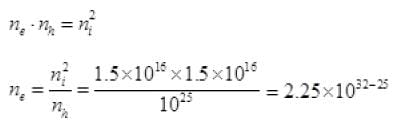=2.25 × 107 electrons per m3
When reversed bias is applied on p-n junction then the minority charge carrier moves toward depletion layer i.e., holes nh=(0.45×1010 per m3) from n side and ne=2.25 × 107/m3 from p side moves towards junction and make the depletion layer thicker.

Q.37 An X-OR gate has following truth table: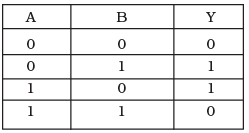It is represented by following logic relation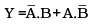Build this gate using AND, OR and NOT gates.

Ans. XOR gate can be realized by the combination of two NOT gates, two AND gates and one OR gate. According to the problem, the logic relation for the . given truth table is
When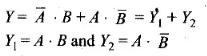Y1 can be obtained as output of AND gate I for which one input is of A through NOT gate and another input is of B. Y2 can be obtained as output of AND gate II for which one input is of A and other input is of B through NOT gate.
Now Y can be obtained as output from OR gate, where Y1, and Y2 are inputs of OR gate.
Thus, the logic circuit of this relation is given below.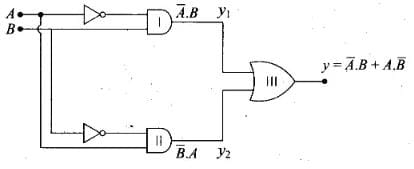Q.38. Consider a box with three terminals on top of it as shown in Figure (a):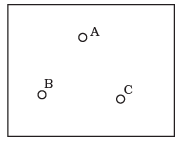(a)

Three components namely, two germanium diodes and one resistor are connected across these three terminals in some arrangement.
A student performs an experiment in which any two of these three terminals are connected in the circuit shown in (b).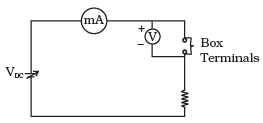(b)The student obtains graphs of current-voltage characteristics for unknown combination of components between the two terminals connected in the circuit.
The graphs are
(i) when A is positive and B is negative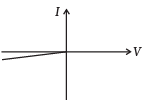(c)(ii) when A is negative and B is positive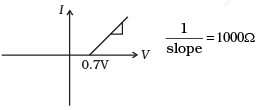(d)(iii) When B is negative and C is positive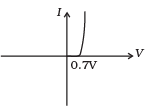(e)(iv) When B is positive and C is negative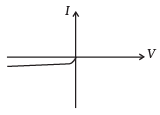(f)(v) When A is positive and C is negative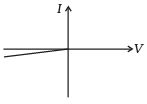(g)(vi) When A is negative and C is positive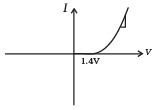(h)From these graphs of current – voltage characteristic shown in Figure (c) to (h), determine the arrangement of components between A, B and C.

Ans. The V-I characteristics of these graph is discussed in points:
(a) In V-I graph of condition (i), a reverse characteristics is shown in figure (c). Here A is connected to n-side of p-n junction I and B is connected top-side of p-n junction I with a resistance in series.
(b) In V-I graph of condition (ii), a forward characteristics is shown in figure (d), where 0.7 V is the knee voltage of p-n junction I. 1/slope = (1/1000) Ω.
It means A is connected to n-side of p-n junction I and B is connected to p-side of p-n junction I and resistance R is in series of p-n junction I between A and B.
(c) In V-I graph of condition (iii), a forward characteristics is shown in figure (e) , where 0.7 V is the knee voltage. In this case p-side of p-n junction II is connected to C and n-side of p-n junction II to B.
(d) In V-I graphs of conditions (iv), (v), (vi) also concludes the above connection of p-n junctions I and II along with a resistance R.
Thus, the arrangement of p-n I, p-n II and resistance R between A, B and C will be as shown in the figure.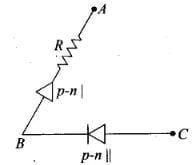Q.39. For the transistor circuit shown in Figure, evaluate VE, RB, RE given IC = 1 mA, VCE = 3V, VBE = 0.5 V and VCC = 12 V, β = 100.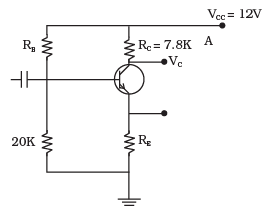Ans. Let us redraw the circuit diagram given here to solve this problem.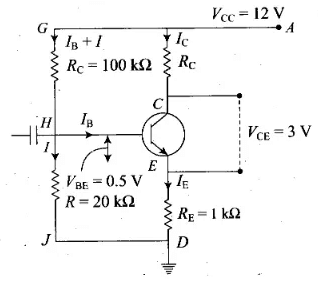As we know the base current is very small. So,
Ic ≈ IE
Rc = 7.8 kΩ
From the figure, IC(RC + RE) + VCE = 12
(RE + RC) x 1 x 10-3 + 3= 1 2
RE + RC = 9 x I03 = 9 k Ω
RE = 9 - 7.8 = 1.2 kΩ
VE = IE x RE
= l x 10-3 x 1.2 x 103 = 1.2 V
Voltage VB = VE +VBE= 1. 2+ 0.5 = 1.7 V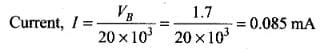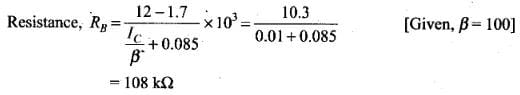Q.40. In the circuit shown in Figure, find the value of RC.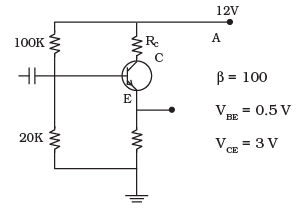Ans. Let us consider the circuit diagram to solve this problem,
IE = IC + IB and IC = βIB     ..(i)
ICRC + VCE + IERE = VCC    ..(ii)
RIB + VBE + IERE = VCC     ..(iii)
∴ IE≈IC = βIB
from (iii)
(R + βRE)IB = VCC - VBE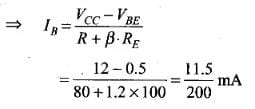from (ii),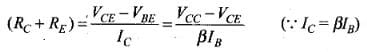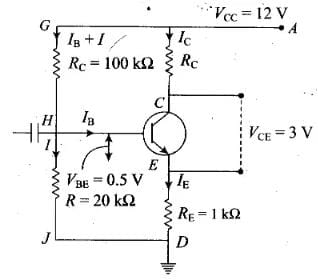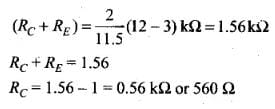The document NCERT Exemplars: Semiconductor Electronics - 2 - Notes | Study Physics Class 12 - NEET is a part of the NEET Course Physics Class 12.
All you need of NEET at this link: NEET

## Physics Class 12

157 videos|452 docs|213 tests
 Use Code STAYHOME200 and get INR 200 additional OFF

## Physics Class 12

157 videos|452 docs|213 tests

### How to Prepare for NEET

Read our guide to prepare for NEET which is created by Toppers & the best Teachers

Track your progress, build streaks, highlight & save important lessons and more!

,

,

,

,

,

,

,

,

,

,

,

,

,

,

,

,

,

,

,

,

,

;